# How to Remove Everything Only Keep Duplicate Rows in Excel

Sometimes we may meet duplicate rows exist in table. In previous tutorial we have taught you how to remove duplicate values in excel, this time we will introduce you the method to only keep duplicate rows in table.

Precondition:

Create a simple table. See screenshot below. Obliviously there are some duplicate values.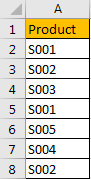## Method: Remove Everything but Only Keep Duplicate Rows by Formula & Filter Function

To look up duplicate values from the list we can apply COUNTIF function here.

Step 1: In cell B2 enter the formula =COUNTIF(\$A\$2:\$A\$8, A2)=1. If data in A list is unique, then formula will return True, otherwise it returns False.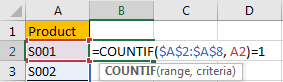Step 2: Click Enter. We get FALSE for product S001, so it has a copy in the list.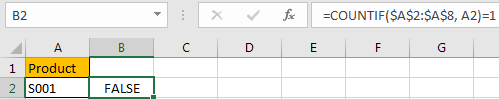Step 3: Drag down the fill handle till reach the end of the list.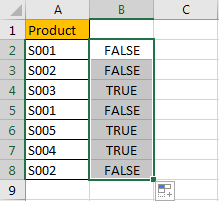Step 4: Click on B column, click Data in ribbon, then click on Filter in Sort & Filter group to create filter.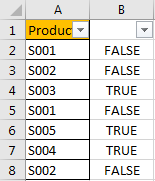Step 5: Click small triangle button to load filter criteria. Check on True under Search section, then click OK.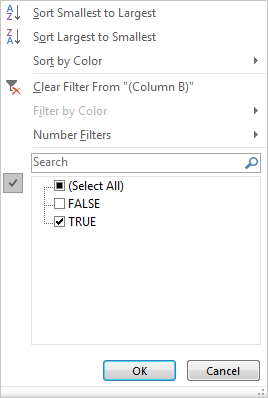Verify that only TRUE values are listed.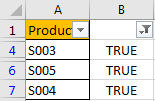Step 6: Select the visible range except headers. Click Home in the ribbon, then click the small triangle belongs to Find & Select icon in Editing group, then select Go To Special option.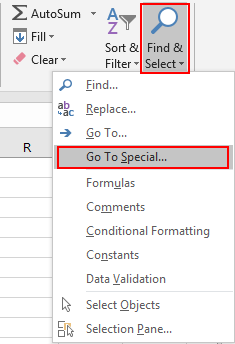Step 7: On Go To Special dialog, check on Visible cells only option, then click OK.Verify that visible cells in the table are selected automatically.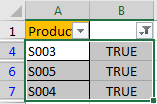Step 8: On selected range, right click to load below options. Select Delete Row.Step 9: On pops up message, click OK. Then entire sheet rows (visible rows) are deleted immediately.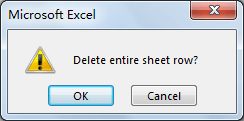Step 10: Click Data->Filter again to clear filter.Verify that only duplicate rows are stayed.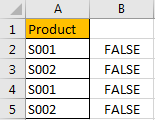Clear texts in B column, then table with only duplicate rows is displayed properly.

Related Posts

Cap percentage values between 0 and 100

This article will talk about how to cap the percentage values between 0% and 100% in Microsoft Excel Spreadsheet or Google Sheets. If you are a newbie on Excel or google Sheets, you may be able to do this by ...

Calculate Cap Percentages to Specific Value

This article will talk about how to limit the cap percentage of a given amount to a given value in Microsoft Excel Spreadsheet or Google Sheets. If you are a newbie on Excel or google Sheets, you may be able ...

Calculate Win Loss Tie

Suppose you got a task to calculate the win, loss, and tie totals; what would you do? If you are new to Ms Excel and don't have enough experience with it, then you might do this task manually but let ...

Calculate Years Between Dates In Ms Excel

If you are an avid Ms Excel user, then you might have come across a task in which you needed to calculate the years between the dates; you might take it easy and do this task manually, which is also ...

Calculate Number of Hours between Two Times

Calculating the difference between two times might be a valuable statistic for subsequent computations or averages, whether you're producing a time sheet for staff or recording personal exercises. While Excel has a plethora of complex functions, including date and time ...

Calculate Loan Interest in Given Year

When you borrow money, you are supposed to repay it gradually. Lenders, on the other hand, want to be compensated for their services and the risk they incur by lending you money. That is, you will not just repay the ...

Calculate Interest Rate for Loan

The interest rate is the fee charged by a lender to a borrower and is expressed as a percentage of the principal—the lent amount. The interest rate on a loan is often expressed as an annual percentage rate, abbreviated as ...

Calculate Interest for Given Period

Using the IPMT function in Excel, we can compute the interest payment on any loan. This step-by-step tutorial will guide Excel users of all skill levels through the process to calculate interest for given period. Finally, the formula: =IPMT(B3/12,1,B5,-B2) The ...

How To Use Excel GCD Function

This post will guide you how to use Excel GCD function with syntax and examples in Microsoft excel. Description The Excel GCD function Returns the greatest common divisor of two or more integers. So you can use the GCD function ...

Calculate A Ratio From Two Numbers In Excel

In elementary mathematics, a ratio is a connection or comparison between two or more integers. For example, ratios are often expressed as ":" to demonstrate the relationship between two numbers. You would think that manually calculating a ratio from two ...

Sidebar